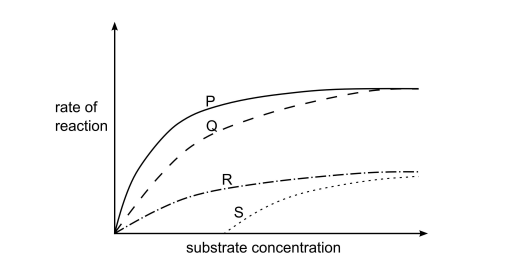# IMAT 2014 Q41 [Enzyme Reactions]

Which curves show the results of calculating the initial rate of reaction of an enzyme:

1. when the substrate concentration is varied, under optimal conditions and without an inhibitor;

2. when the substrate concentration is varied, under optimal conditions and fixed, low concentration of a competitive inhibitor?A. (1) curve P; (2) curve S
B. (1) curve R; (2) curve P
C. (1) curve Q; (2) curve R
D. (1) curve P; (2) curve Q
E. (1) curve R; (2) curve S

We can discuss the curve of statement (1):

As we increase the substrate concentration, we should see a constant increase in the rate of reaction as long as there is substrate available to bind to. This therefore, should look like curve P.

With competitive inhibitors, we must know that the end result reached under optimal conditions and the same substrate concentration, is the maximum rate of reaction still being reached. The difference is, is that the reaction should take longer because of this “competition”. In other words, the rate of the reaction should be slower.

Therefore for statement (2), we must choose an option in which the rate of reaction is lower, but we still reach it’s maximum. This should be option Q.